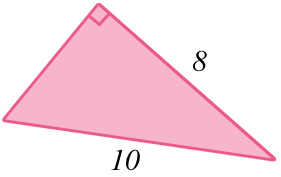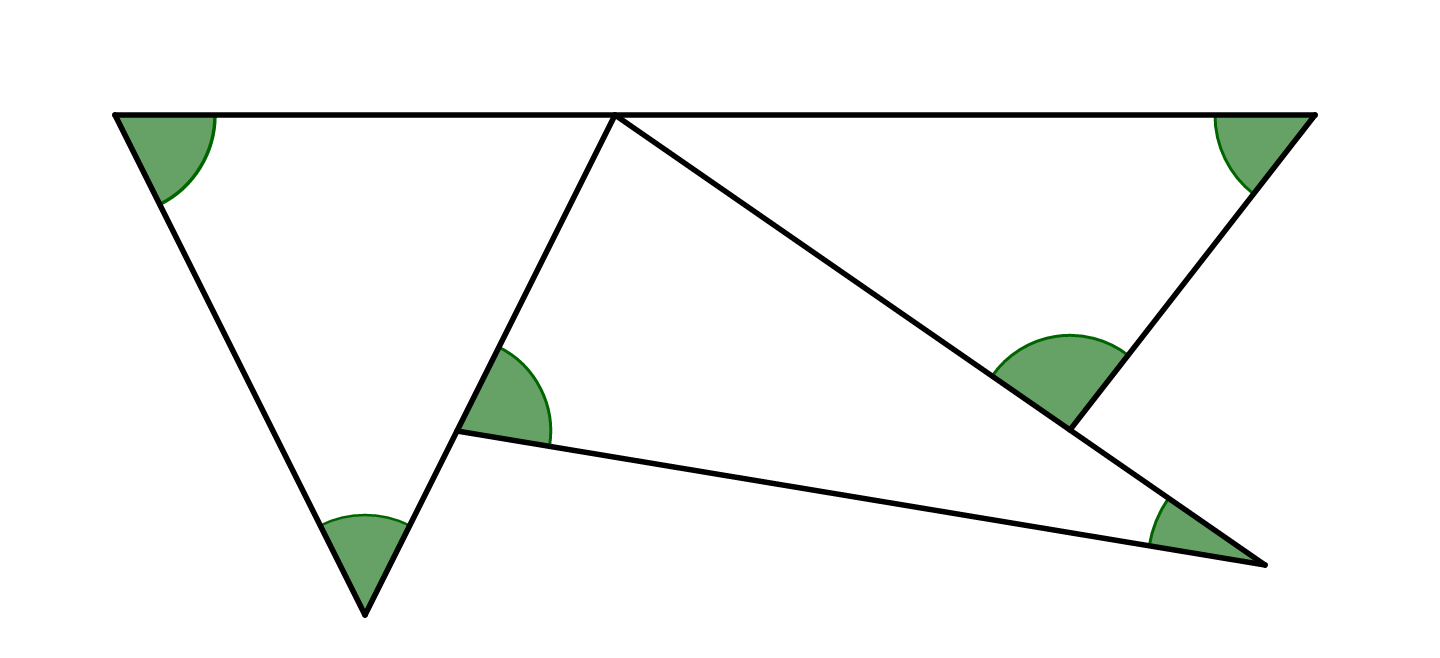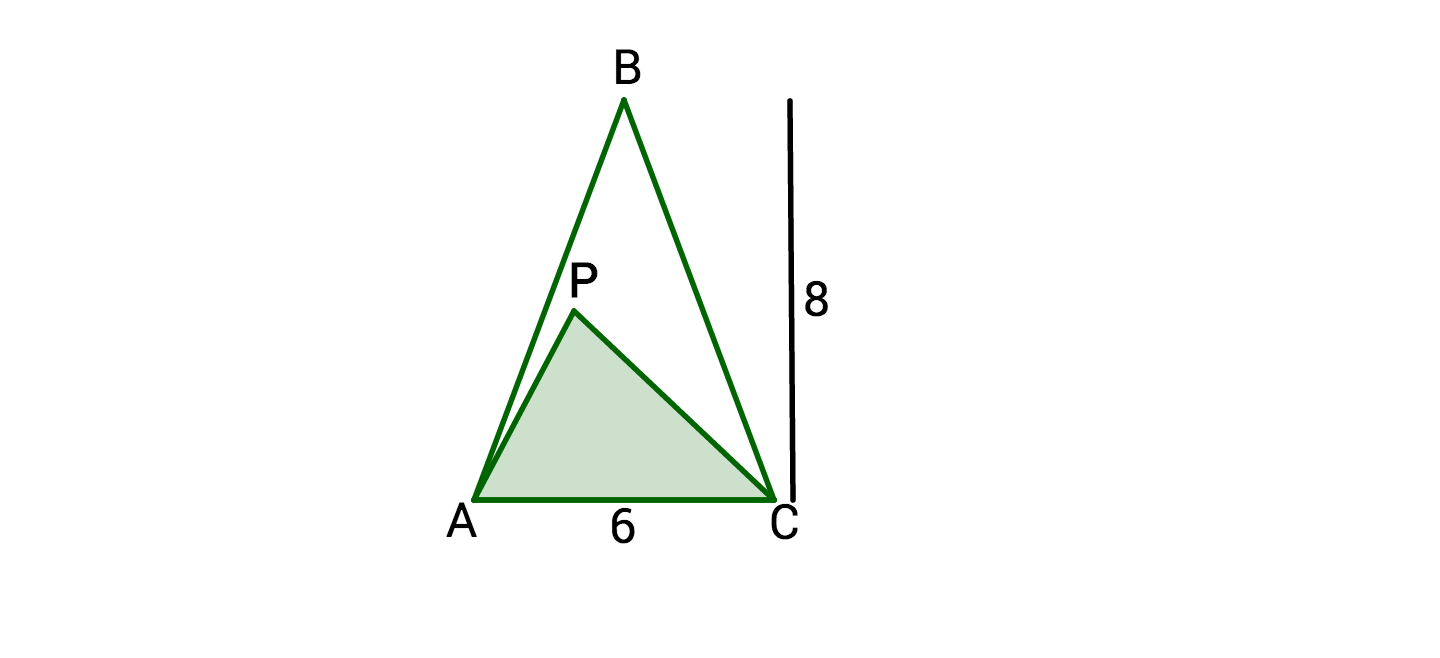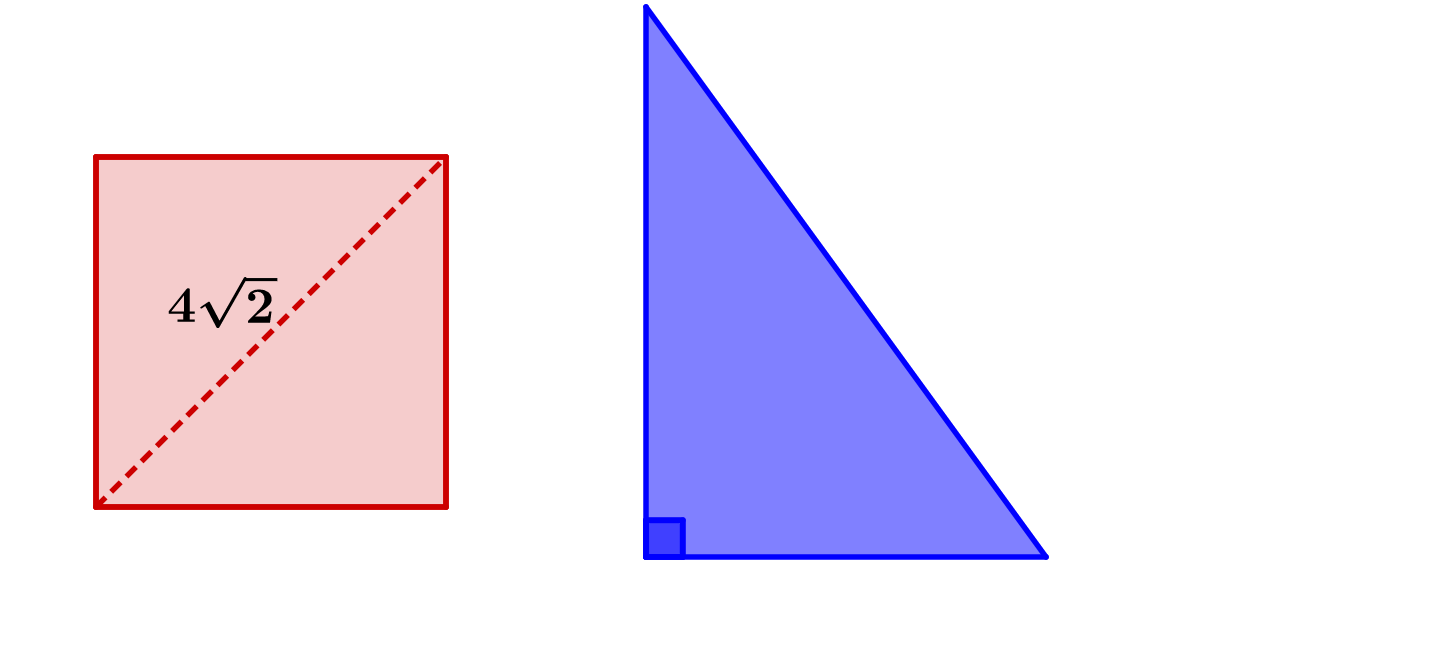Geometry

# Properties of Triangles WarmupWhat is the area of the triangle?What is the sum of the angles shaded in green?If the height of $\triangle APC$ is 4, what percent of $\triangle ABC$ is shaded white?If a square with a diagonal length of $4\sqrt{2}$ has the same area as a right triangle, what could be the base and height of the triangle?

If a right triangle has two side lengths of 1 and 2, which of these options couldn't be the length of the third side?

×